## 桶排序（Bucket sort）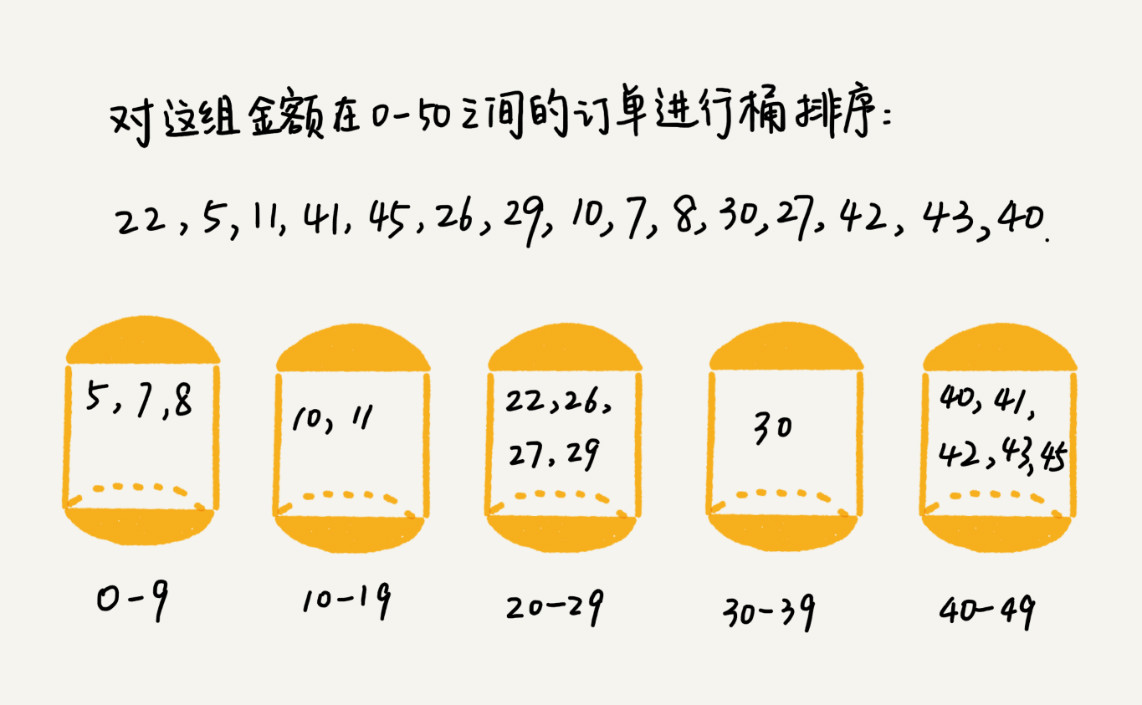## 计数排序（Counting sort）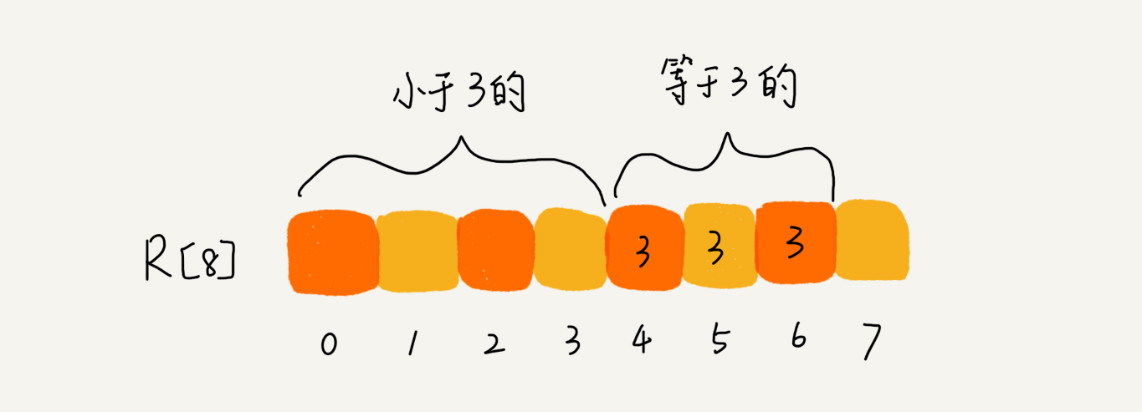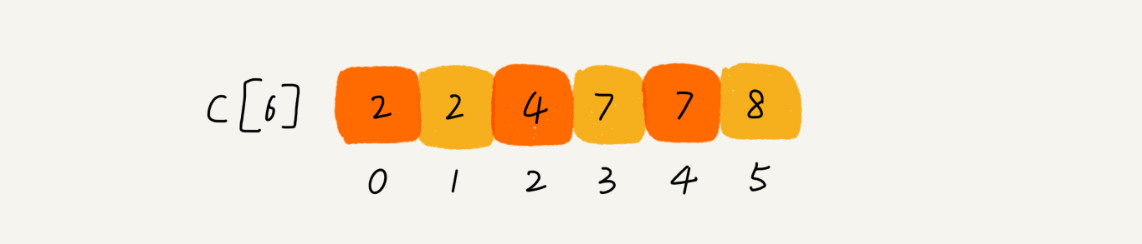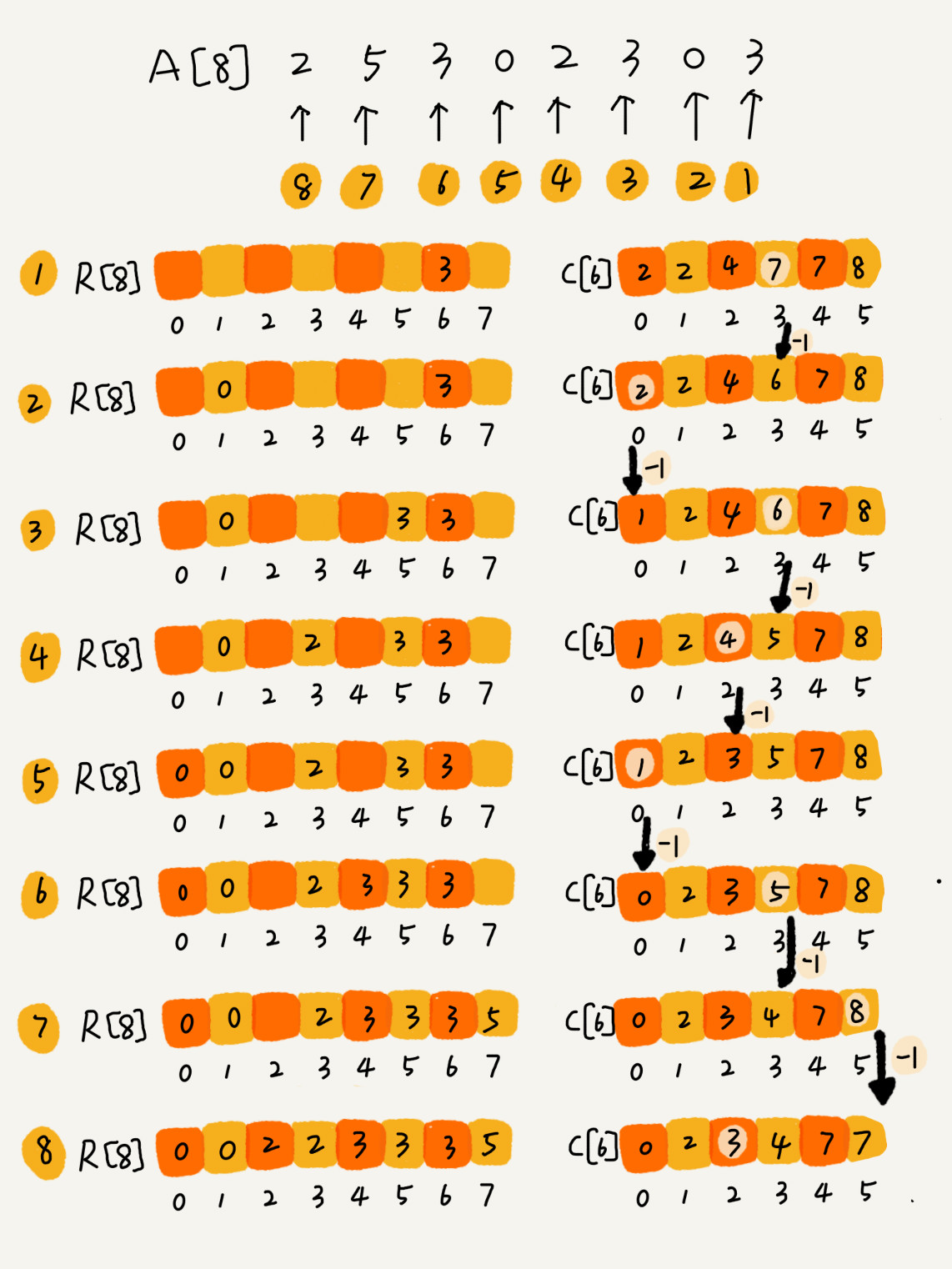// 计数排序，a 是数组，n 是数组大小。假设数组中存储的都是非负整数。
public void countingSort(int[] a, int n) {
if (n <= 1) return;

// 查找数组中数据的范围
int max = a;
for (int i = 1; i < n; ++i) {
if (max < a[i]) {
max = a[i];
}
}

int[] c = new int[max + 1]; // 申请一个计数数组 c，下标大小 [0,max]
for (int i = 0; i <= max; ++i) {
c[i] = 0;
}

// 计算每个元素的个数，放入 c 中
for (int i = 0; i < n; ++i) {
c[a[i]]++;
}

// 依次累加
for (int i = 1; i <= max; ++i) {
c[i] = c[i-1] + c[i];
}

// 临时数组 r，存储排序之后的结果
int[] r = new int[n];
// 计算排序的关键步骤，有点难理解
for (int i = n - 1; i >= 0; --i) {
int index = c[a[i]]-1;
r[index] = a[i];
c[a[i]]--;
}

// 将结果拷贝给 a 数组
for (int i = 0; i < n; ++i) {
a[i] = r[i];
}
}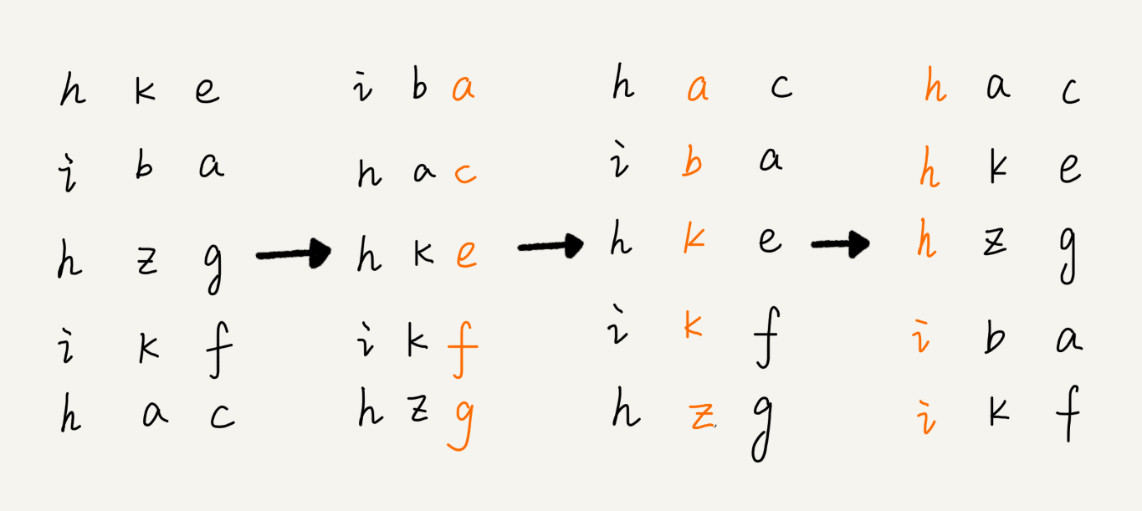## 五、思考

• 如何根据年龄给100万用户数据排序？

实际上，根据年龄给 100 万用户排序，就类似按照成绩给 50 万考生排序。假设年龄的范围最小 1 岁，最大不超过 120 岁。
可以遍历这 100 万用户，根据年龄将其划分到这 120 个桶里，然后依次顺序遍历这 120 个桶中的元素。
这样就得到了按照年龄排序的 100 万用户数据。

• D，a，F，B，c，A，z这几个字符串进行排序，要求将其中所有小写字母都排在大写字母前面，但是小写字母内部和大写字母内部不要求有序。比如经过排序后为a，c，z，D，F，B，A，这个如何实现呢？如果字符串中处理大小写，还有数字，将数字放在最前面，又该如何解决呢？

用两个指针a、b
a指针从头开始往后遍历，遇到大写字母就停下，b从后往前遍历，遇到小写字母就停下，交换a、b指针对应的元素；
重复如上过程，直到a、b指针相交。
对于小写字母放前面，数字放中间，大写字母放后面，可以先将数据分为小写字母和非小写字母两大类，进行如上交换后再在非小写字母区间内分为数字和大写字母做同样处理